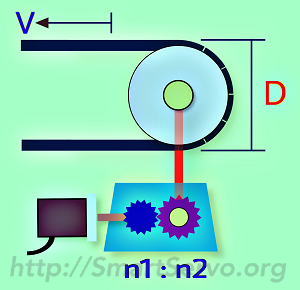# Electronic gear ratio (on-line) calculator – Belt mechanism

This article provides an online calculator for the Belt or Roller Mechanism to quickly find the servo’s electronic gear ratio and provide additional simulation information to evaluate whether the system parameters meet the requirements. The steps are as follows:

1. Define  eg, How many PUUs correspond to 1 mm
2. Enter the mechanical Reduction ratio (1:1 without deceleration)
3. Enter the diameter(D) or circumference of the pully, including the belt.
4. Enter Encoder Resolution(PLS), PLS number per turn of the Encoder,ie,when the electronic gear ratio is 1:1, how many PUUs the drive has to receive in order to rotate 1 turn.
5. Press the “Calculate” button to get the numerator and denominator of the E-Gear Ratio
6. Select “Significant digit number“: to specify the numeric width of the numerator,  suggest 6 or above [Note 1].
7. Enter the Line Speed(​​V) of the machine to check if the simulation results meet the requirements ?﻿

Input Data： Define PUU  ： = PUU（Pos of User Unit）

Reduction Ratio ：n1 =   ： n2 = 　Note：n1 <= n2 for deceleration

Pulley/Roller Diameter =  mm， or circumference = mm

Encoder's Resolution = （PLS/rev）PLS number per turn of the Encoder

Significant digits：

Result：Electronic Gear Ratio　 Num = ：Den =
Simulation infomation：

Line speed of the machine V ＝ （mm/min）or   （mm/sec）

Results： Motor Speed ＝   rpm，Must not exceed the maximum speed of the motor

Command pulse Freq. ＝   kpps(KPUU per Sec)，Must < PLC output capability

PUUs per turn of motor ＝  PUU/rev ，recommended 　＞　5000

formula：circumference(PUU) x Num／Den = R(PLS/Rev) x N2／N1

The simulation information will be displayed when the line speed of the machine is entered. The description of each field is as follows :

1. Motor speed : Should not exceed the maximum speed of the motor, otherwise the reduction ratio of the machine must be reduced or the diameter of the pulley or roller should be increased.
2. Command Pulse frequency : Must be less than the highest output pulse frequency of the controller (or PLC), otherwise the required line speed cannot be reached. If it is exceeded, please modify the PUU unit to increase the electronic gear ratio ! This message can be ignored if the command comes from the drive itself (eg Delta ASD-A2 Smart Servo)
3. PUUs per turn of motor : It is recommended that this value not be less than 5000 to avoid jitter when the motor rotates. The lower the speed, the more obvious! Reducing the electronic gear ratio can improve this.

For the formula of this calculator, please refer to the article “Electronic Gear Ratio Formula Derivation” and replace the Pitch into the circumference of the pulley or roller.

Ref. E-Gear ratio calculator – for Indexer/Turret

[Note 1] Since the calculation of the circumference of the roller includes the pi, the irrational number, cannot be converted into an accurate numerator/denominator form, and only an approximation can be obtained. Therefore, the option “Significant digit number” is provided for the user to select according to the demand or the data width of the drive!